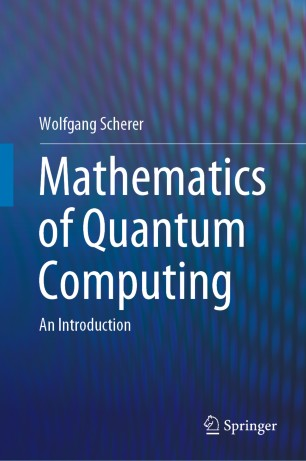# Mathematics of Quantum Computing

## An Introduction

• Wolfgang SchererTextbook

1. Front Matter
Pages i-xix
2. Wolfgang Scherer
Pages 1-9
3. Wolfgang Scherer
Pages 11-75
4. Wolfgang Scherer
Pages 77-125
5. Wolfgang Scherer
Pages 127-160
6. Wolfgang Scherer
Pages 161-246
7. Wolfgang Scherer
Pages 247-341
8. Wolfgang Scherer
Pages 343-402
9. Wolfgang Scherer
Pages 403-497
10. Wolfgang Scherer
Pages 499-500
11. Back Matter
Pages 501-764

### Introduction

This textbook presents the elementary aspects of quantum computing in a mathematical form. It is intended as core or supplementary reading for physicists, mathematicians, and computer scientists taking a first course on quantum computing. It starts by introducing the basic mathematics required for quantum mechanics, and then goes on to present, in detail, the notions of quantum mechanics, entanglement, quantum gates, and quantum algorithms, of which Shor's factorisation and Grover's search algorithm are discussed extensively. In addition, the algorithms for the Abelian Hidden Subgroup  and Discrete Logarithm problems are presented and the latter is used to show how the Bitcoin digital signature may be compromised. It also addresses the problem of error correction as well as giving a detailed exposition of adiabatic quantum computing. The book contains around 140 exercises for the student, covering all of the topics treated, together with an appendix of solutions.

### Keywords

Quantum computing math Entanglement measures Bell inequality Quantum algorithms Shor factorization algorithm Grover search algorithm Phase Estimation Search for Hidden Subgroup Quantum error correction Quantum computing textbook Textnook quantum computing Text quantum information

#### Authors and affiliations

• Wolfgang Scherer
• 1
1. 1.KingstonUK